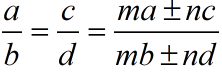Monday, September 27, 2021
Home > ICSE Solutions for Class 10 Maths > Ratio and Proportion Class 10 ICSE Maths Revision Notes Chapter 6 PDF

# Ratio and Proportion Class 10 ICSE Maths Revision Notes Chapter 6 PDFHi students, Welcome to Amans Maths Blogs (AMB). In this article, you will get Ratio and Proportion ICSE Class 10 Maths Revision Notes & Solutions Chapter 6 PDF.

## Ratio and Proportion ICSE Class 10 Math Revision Notes

### Ratio

Ratio is a value obtained when two quantities of the same kind are compared by dividing one quantity with the other. The ratio of two quantities is the value of which gives us how many times or how many parts one quantity is of the other.

The ratio of two quantity a and b is written as a : b or a/b. If two quantities are in ratio a : b, then first quantity is a/(a + b) times of the sum of the quantities and the second quantity is b/(a + b) of the sum of the quantities.

### Proportion

If four quantities a, b, c and d are such that a : b = c : d or a/b = c/d, then the four quantities a, b, c and d are in proportion. It is written as a : b : : c : d or a : b = c : d.

In this four quantities of proportion a : b : : c : d, the first and fourth terms (i.e., a and d) are called extremes and the second and third terms (i.e., b and c) are called means.

When four quantities are in proportion, the product of extremes and the product of means.

If four quantities a, b, c and d are in proportion, then

a : b = c : d ⇒ a/b = c/d ⇒ ad = bc ⇒ Product of Extremes = Product of Means.

### Continued Proportion

If three quantities a, b, c are in continued proportion, then a : b = b : c

a/b = b/cb2 = ac ⇒ b = √ac. Here, b is called mean proportional between a and c.

c are called third proportional to a and b.

If four quantities a, b, c, d are in continued proportion, then a : b = b : c = c : d.

(i) In a : b = c : d, if a = c, then b = d and if b = d, then a = c.

(ii) If a : b = c : d, then b : a = d : c.

It means, if four quantities are proportion, then their reciprocals are also in proportion.

This property is known as INVERTENDO.

(iii) If a : b = c : d, then a : c = b : d.

It means, if four quantities are proportion, then their alternatives are also in proportion.

This property is known as ALTERTENDO.

(iii) If a : b = c : d, then a + b : b = c + d : d.

It means, if four quantities are proportion, then first together with the second is to the second as third together with the fourth is to the fourth.

This property is known as COMPONENDO.

(iv) If a : b = c : d, then a – b : b = c – d : d.

It means, if four quantities are proportion, then excess of the first over the second is to the second as the excess of the third over the fourth to the fourth.

This property is known as DIVIDENDO.

(iv) If a : b = c : d, then a + b : a – b = c + d : c – d.

It means, if four quantities are proportion, then the sum of first and the second is to their difference as the sum of the third and fourth is to their difference.

This property is known as COMPONENDO and DIVIDENDO.

### k-Method in Proportion

(i) If a/b = c/d = k, then a = bk and c = dk. Hence,Get Online Maths Tuition by AMBiPi for Class 8 to 12